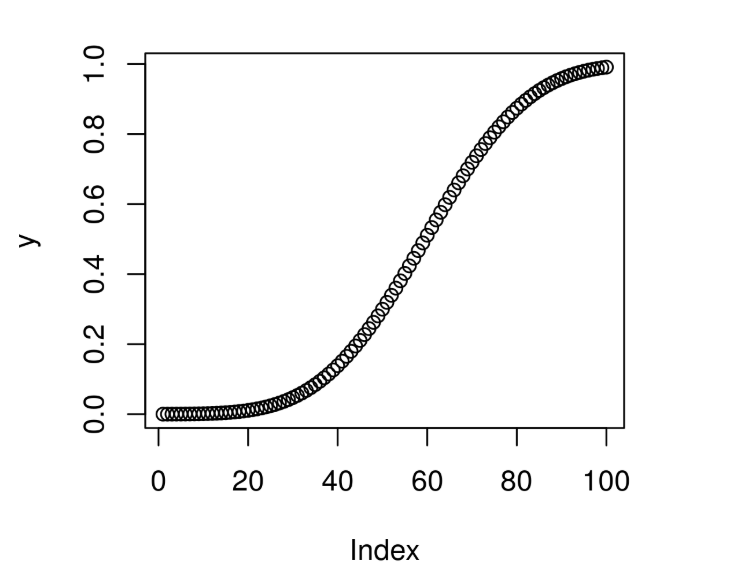# Compute the value of CDF over Wilcoxon Signedrank Distribution in R Programming – psignrank() Function

`psignrank()` function in R Language is used to compute the value of Cumulative Density Function(CDF) over Wilcoxon Signedrank Statistic Distribution for a sequence of Numeric values.

Syntax: psignrank(x, n)

Parameters:
x: Numeric Vector
n: Sample Size

Example 1:

 `# R Program to compute the value of ` `# CDF over Wilcoxon Signedrank Distribution ` ` `  `# Creating a sequence of x-values ` `x <``-` `seq(``0``, ``20``, by ``=` `1``) ` ` `  `# Calling psignrank() Function ` `y <``-` `psignrank(x, n ``=` `10``) ` `y `

Output:

```  0.0009765625 0.0019531250 0.0029296875 0.0048828125 0.0068359375
 0.0097656250 0.0136718750 0.0185546875 0.0244140625 0.0322265625
 0.0419921875 0.0527343750 0.0654296875 0.0800781250 0.0966796875
 0.1162109375 0.1376953125 0.1611328125 0.1875000000 0.2158203125
 0.2460937500
```

Example 2:

 `# R Program to compute the value of ` `# CDF over Wilcoxon Signedrank Distribution ` ` `  `# Creating a sequence of x-values ` `x <``-` `seq(``1``, ``100``, by ``=` `1``) ` ` `  `# Calling psignrank() Function ` `y <``-` `psignrank(x, n ``=` `15``) ` ` `  `# Plot a graph ` `plot(y) `

Output:My Personal Notes arrow_drop_upCheck out this Author's contributed articles.

If you like GeeksforGeeks and would like to contribute, you can also write an article using contribute.geeksforgeeks.org or mail your article to contribute@geeksforgeeks.org. See your article appearing on the GeeksforGeeks main page and help other Geeks.

Please Improve this article if you find anything incorrect by clicking on the "Improve Article" button below.

Article Tags :

Be the First to upvote.

Please write to us at contribute@geeksforgeeks.org to report any issue with the above content.